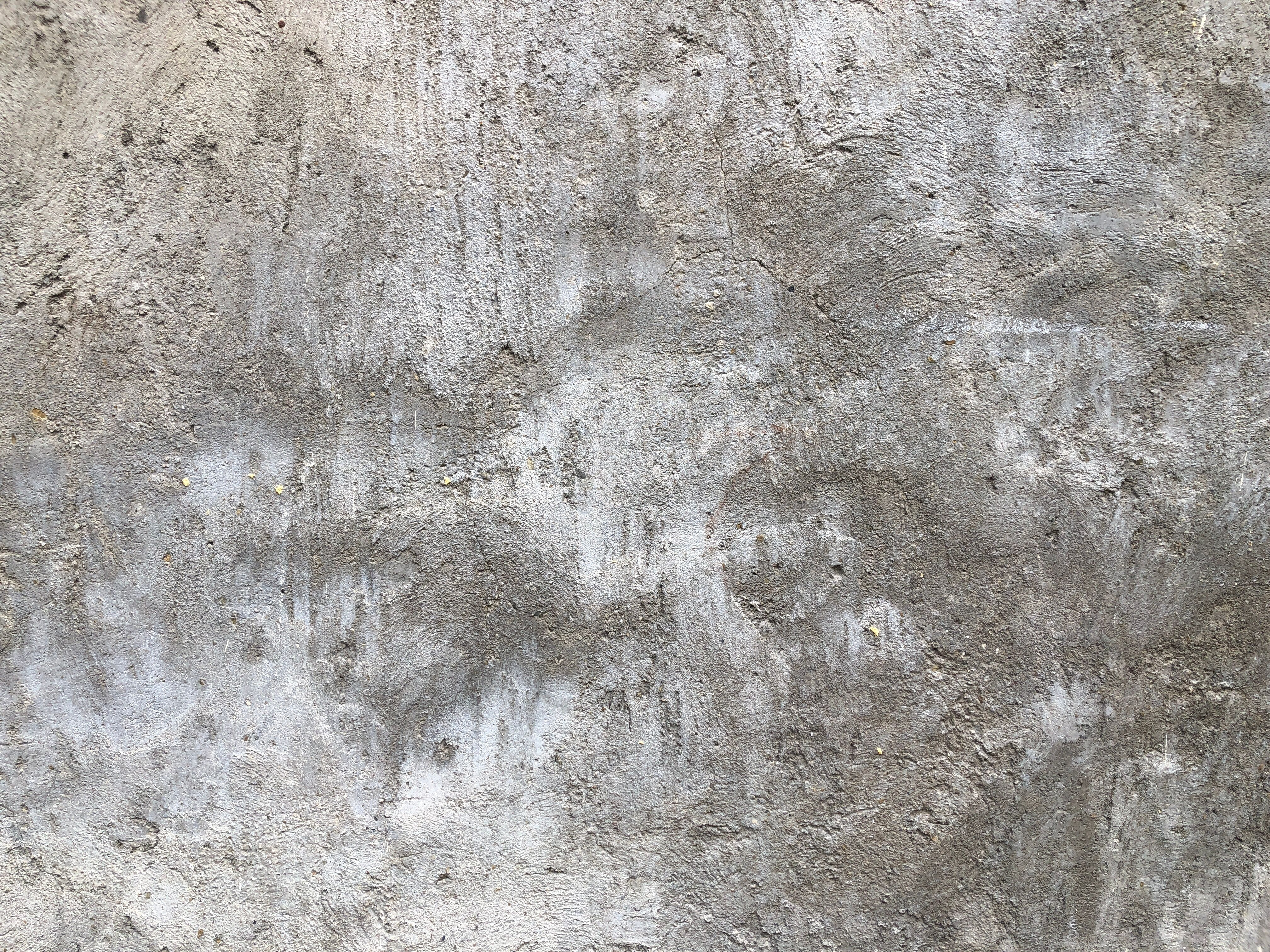# Is Calc 1 or 2 harder?

Is Calc 1 or 2 harder? – College Tips and Tricks

# Is Calc 1 or 2 harder?Calculus is one of the most difficult mathematical courses and is considered a rite of passage for students pursuing science, technology, engineering, and mathematics (STEM) fields. Students often face challenges when it comes to determining which of the calculus sequences – Calculus 1 (Calc 1) or Calculus 2 (Calc 2) – is harder. As you might expect, students tend to have more difficulty with Calc 2 than Calc 1. While Calc 1 is mostly about limits and differentiation, Calc 2 also covers some of the fundamental theorems for Calc 3. Achieving success requires stronger math skills and a solid understanding of the subject matter.

## FAQs

### 1. What is Calc 1?

Calc 1, also known as Differential Calculus, is the first course in the calculus sequence. It covers the basics of calculus, including limits, derivatives, and applications of derivatives such as optimization problems, related rates, and curve sketching. Calc 1 is generally considered to be an easier course than Calc 2.### 2. What is Calc 2?

Calc 2, also known as Integral Calculus, is the second course in the calculus sequence. It builds on the concepts from Calc 1 and covers integration, applications of integration such as area and volume problems, techniques of integration, series, and polar coordinates. Calc 2 is generally considered to be a more difficult course than Calc 1 due to the complexity of the concepts covered and the need for a more comprehensive understanding of calculus.

### 3. What makes Calc 2 harder than Calc 1?

Calc 2 is harder than Calc 1 because it requires a deeper understanding of calculus concepts and their applications. It also involves more complex integration techniques, such as substitution, integration by parts, and partial fractions. Calc 2 also heavily relies on knowledge of algebra and trigonometry, making it more challenging than Calc 1, which is mostly focused on differentiation.### 4. How can I succeed in Calc 2?

To succeed in Calc 2, you need to have a solid understanding of the concepts covered in Calc 1. You should also develop strong algebra and trigonometry skills, as well as basic calculus skills such as differentiation and integration. Practice is key to success in Calc 2. You should work on practice problems and seek help from professors or tutors when necessary. Additionally, using online resources and textbooks can supplement your learning outside of the classroom.

## Conclusion

Calc 1 and Calc 2 are both challenging courses that require strong math skills and a solid understanding of calculus. While Calc 1 is generally considered to be an easier course, Calc 2 is more difficult due to its complexity and reliance on knowledge of algebra and trigonometry. However, with dedication, practice, and the right resources, students can succeed in both Calc 1 and Calc 2.